# Split Text and Numbers in Excel

This post explains how to split text and numbers in excel, or how to extract the text characters from another strings with text and numbers combined.

## Split Text and Numbers

If you want to split text and numbers, you can run the following excel formula that use the MIN function, FIND function and the LEN function within the LEFT function in excel. And if you want to extract only numbers within string, then you also need to RIGHT function to combine with the above functions.

`=LEFT(MIN(FIND({0,1,2,3,4,5,6,7,8,9},B1&"0123456789")))`

To split the text and numbers, the key is to get the position of the first digit within the Cell B1, once the position of the first number is located, you can use the LEFT function or RIGHT function to extract text characters or just numbers.

Let’s see how the above formula works as below:

To get the position of the first number with the cell B1, and you can use the following formula:

`=MIN(FIND({0,1,2,3,4,5,6,7,8,9},B1&"0123456789"))`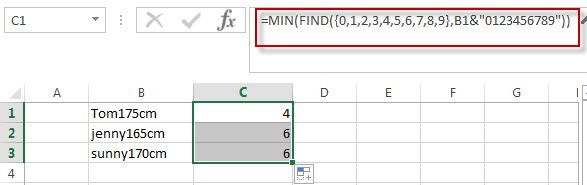The position of the first number is returned from the above Excel formula.

The Find function will locate the starting position of the first number within the cell B1. For the second argument in FIND function, the array constant is used, and the find function will use this array constant to perform a separate search for each number in the array.  And then the returned value of the FIND function also will be an array that contains the position of all numbers in string. The MIN function will use the returned array value of the FIND function to get the minimal value, it is the position of the first number within the Cell B1.

To extract text characters within the Cell B1, using the following formula:

`=LEFT(B1, MIN(FIND({0,1,2,3,4,5,6,7,8,9},B1&"0123456789"))-1)`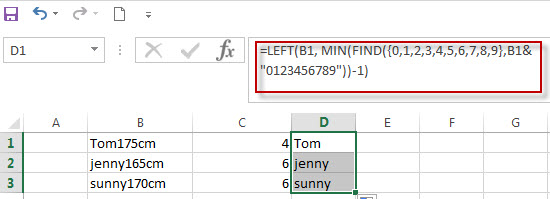To extract numbers within the cell B1, using the following formula:

`=RIGHT(B1,LEN(B1)- MIN(FIND({0,1,2,3,4,5,6,7,8,9},B1&"0123456789"))+1)`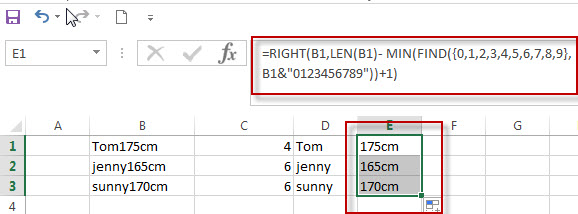The above formula is only works for the string of “text+number” pattern.

## Split String with Numbers and Text combined (Number+Text)

If you need to split the string that the text characters appears after number, the key is to get the length of all numbers left to of the text characters in the Cell B1.  Just using the following formula:

`= SUM(LEN(B1) - LEN(SUBSTITUTE(B1, {0,1,2,3,4,5,6,7,8,9}, "")))`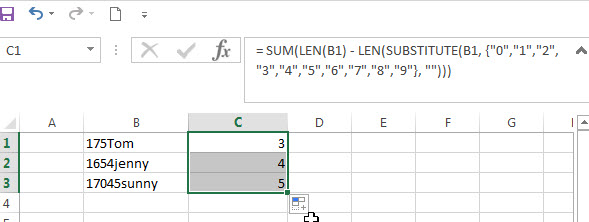In the above formula, the SUBSTITUTE function will use the array constant {0,1,2,3,4,5,6,7,8,9}, it will perform a separate replace for each value in the array constant with new_text (empty string). Then it will return another array with 10 values.  And the second LEN function will use the returned array values of SUBSTITUTE function to get the length of each string. The First LEN function will return the length of the cell B1. The SUM function will calculate the length of numbers with in Cell B1.

To extract numbers within cell B1, using the following formula:

`=LEFT(B1,SUM(LEN(B1)-LEN(SUBSTITUTE(B1,{0,1,2,3,4,5,6,7,8,9},""))))`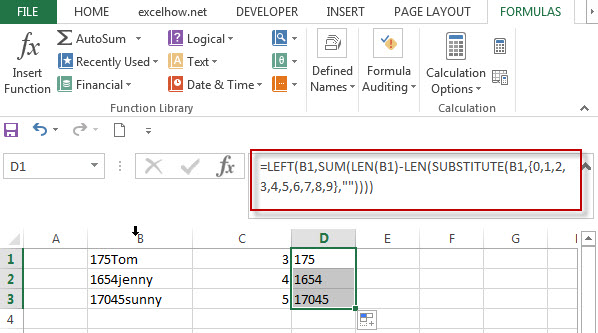To extract text within Cell B1, using the following formula:

`=RIGHT(B1,LEN(B1)- SUM(LEN(B1)-LEN(SUBSTITUTE(B1, {0,1,2,3,4,5,6,7,8,9}, ""))))`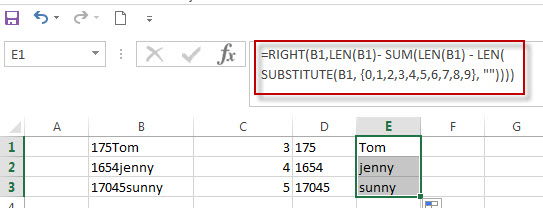### Related Formulas

• Split Text String by Specified Character in Excel
you should use the Left function in combination with FIND function to split the text string in excel. And we can refer to the below formula that will find the position of “-“in Cell B1 and extract all the characters to the left of the dash character “-“.=LEFT(B1,FIND(“-“,B1,1)-1).…
• Split Multiple Lines from a Cell into Rows
If you have multiple lines in a cell and each line is separated by line break character or press “alt + enter” key while you entering the text string into cells, and you need to extract the multiple lines into the separated rows or columns, you can use a combination with the TRIM function, the MID function, the SUBSTITUTE function, the REPT function, the LEN function to create a complex excel formula..…
• Split Text String by Line Break in Excel
When you want to split text string by line break in excel, you should use a combination of the LEFT, RIGHT, CHAR, LEN and FIND functions. The CHAR (10) function will return the line break character, the FIND function will use the returned value of the CHAR function as the first argument to locate the position of the line break character within the cell B1.…
• Split Text and Numbers
If you want to split text and numbers, you can run the following excel formula that use the MIN function, FIND function and the LEN function within the LEFT function in excel. And if you want to extract only numbers within string, then you also need to RIGHT function to combine with the above functions..…

### Related Functions

• Excel Find function
The Excel FIND function returns the position of the first text string (substring) from the first character of the second text string.The FIND function is a build-in function in Microsoft Excel and it is categorized as a Text Function.The syntax of the FIND function is as below:= FIND  (find_text, within_text,[start_num])…
• Excel SUBSTITUTE function
The Excel SUBSTITUTE function replaces a new text string for an old text string in a text string.The syntax of the SUBSTITUTE function is as below:= SUBSTITUTE  (text, old_text, new_text,[instance_num])…
• Excel LEFT function
The Excel LEFT function returns a substring (a specified number of the characters) from a text string, starting from the leftmost character.The LEFT function is a build-in function in Microsoft Excel and it is categorized as a Text Function.The syntax of the LEFT function is as below:= LEFT(text,[num_chars])…
• Excel LEN function
The Excel LEN function returns the length of a text string (the number of characters in a text string).The LEN function is a build-in function in Microsoft Excel and it is categorized as a Text Function.The syntax of the LEN function is as below:= LEN(text)…
• Excel MIN function
The Excel MIN function returns the smallest numeric value from the numbers that you provided. Or returns the smallest value in the array.The syntax of the MIN function is as below:= MIN(num1,[num2,…numn]
• Excel RIGHT function
The Excel RIGHT function returns a substring (a specified number of the characters) from a text string, starting from the rightmost character.The syntax of the RIGHT function is as below:= RIGHT (text,[num_chars])…
• Excel SUM function
The Excel SUM function will adds all numbers in a range of cells and returns the sum of these values. You can add individual values, cell references or ranges in excel.The syntax of the SUM function is as below:= SUM(number1,[number2],…)…